### Device Purposes

The optimization of a semiconductor device often requires to perform calibrations and parameter extractions for certain models where reference data is available either through measurements or Monte Carlo (MC) simulations [278,279]. In general, the optimization for device simulation purposes requires to modify certain model parameters to match the simulated characteristics with reference data. This fact offers the possibility to tune certain local optimizer tools to operate at vectorized target quantities as for instance the LEVENBERG-MARQUARDT algorithms does using an norm to transform the vector-valued quantity to a scalar-valued one. As an alternative the score function can be extended by a p-norm operator to transform a vector-valued quantity into a representative scalar-valued quantity. However, with increasing numbers of model parameter to tune, the optimization task becomes more complex in terms of the parameter dimensions and the number of required parameter evaluations can often demand a tremendous effort if the evaluation of the parameter is computationally expensive. Nevertheless, gradient-based optimization algorithms and heuristic approaches like simulated annealing serve well for a wide range of problem classes and deliver reasonable and accurate results [280,281].

However, optimization in which the ranges of the parameters differ by orders of magnitude face other challenges. If certain parameters in the range [0,1] have to be considered as well as doping concentration in the range of [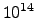,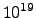], the numerical precision is often not sufficient to compute the parameter correctly. Therefore, the latter parameter can be manually transformed either to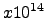withor to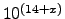with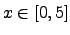. This particular problem can often be solved by a rigorous scaling of the optimization software. However, each numerical challenge requires a program to solve the problem with respect to the underlying simulation software because the program has to clarify if this simple parameter transformation can be applied to a simulation tool. If not, an additional program is required to produce the appropriate input format for the simulator.

Stefan Holzer 2007-11-19